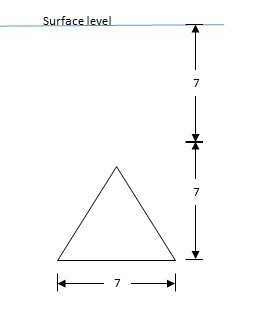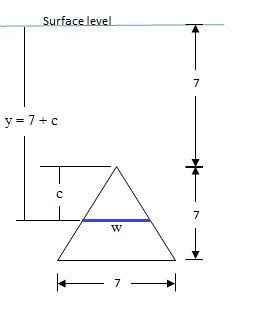Find the fluid force exerted against the vertically submerged flat surface depicted in the...

Question:

Find the fluid force exerted against the vertically submerged flat surface depicted in the diagram. Assume arbitrary units, and call "W" the weight-density of the fluid.Hydrostatic Force:

The hydrostatic force exerted by a fluid on a horizontal body is given by the equation:

$$F = P \times A$$

where {eq}P = \rho g h {/eq} is the hydrostatic pressure on the horizontal body at same depth {eq}h {/eq}, {eq}\rho {/eq} is the fluid density and {eq}g {/eq} is the acceleration due to gravity.

Given a plate of height {eq}H {/eq} that is fully submerged in a fluid such that one of its sides is touching the surface of the water. Let the width of the plate {eq}w(y) {/eq} be a function of the depth {eq}y {/eq}, assuming the positive y-direction is downwards.

The total hydrostatic force exerted by the fluid on the plate is the sum of all the forces exerted on thin horizontal slices of the plate and is given by the integral:

\begin{align*} &F = \int_0^H P\,dA = \int_0^H \rho g y\, w(y) \,dy & \text{[Hydrostatic Force]} \end{align*}

In general, suppose the topmost side of the plate is submerged underwater at a depth of {eq}H_0 {/eq}. The hydrostatic force exerted by the fluid on the plate is given by the integral:

$$F = \int_{H_0}^{H_0+H} P\,dA = \int_{H_0}^{H_0+H} \rho g y\, w(y) \,dy$$

Given the triangular flat surface with base and height equal to {eq}H = 7 {/eq} submerged vertically such that the topmost vertex is {eq}H_0 = 7 {/eq} units below the surface.

Let us define the positive y-direction to be downwards, and the origin to be at the water surface directly above the topmost vertex of the triangular surface.

Additionally, at any depth {eq}y {/eq}, let {eq}w {/eq} be the width of the flat surface as shown below:Based on the diagram, at any depth from {eq}y \in [7,14] {/eq}, the width of the flat surface can be expressed in terms of the depth as:

{eq}\begin{align*} &\frac{w}{7} = \frac{c}{7} & \text{[Similar Triangles]} \\ &\Rightarrow w = c & \text{[Substitute } c = y - 7 ] \\ &\Rightarrow w = y - 7 & \text{[Width of the Flat Surface in terms of the Depth]} \end{align*} {/eq}

Therefore, the fluid force exerted against the flat surface is given by the integral:

{eq}\begin{align*} Force &= \int_7^{14} yW ( y - 7)\,dy & \text{[Where the weight density of the fluid is } W = \rho g ] \\ &= W \int_7^{14} (y^2 - 7y) \,dy & \text{[Integrate with respect to } y \text{]} \\ &= W \left. \left( \frac{y^3}{3} - \frac{7y^2}{2} \right) \right|_7^{14} & \text{[Fundamental Theorem of Calculus]} \\ &= W \left( \left[ \frac{14^3}{3} - \frac{7(14)^2}{2} \right] - \left[ \frac{7^3}{3} - \frac{7(7)^2}{2} \right] \right) \\ &= W \left( \frac{686}{3} + \frac{343}{6} \right) \\ Force &= \boxed{ \frac{1715W}{6} \approx 285.833 W } & \boxed{ \text{Force Exerted on the Vertically Submerged Triangular Flat Surface} } \end{align*} {/eq}